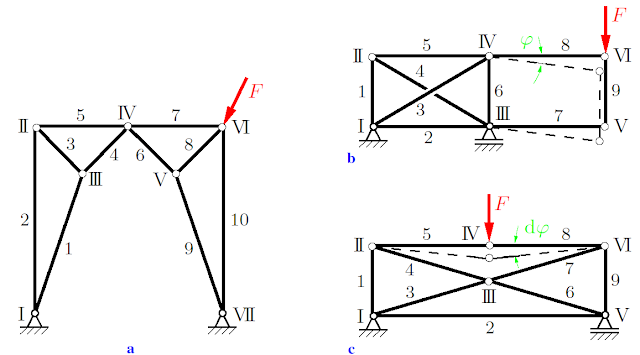# Statically Determinate Trusses

A truss is a structure composed of slender members that are connected at their ends by joints. The truss is  one of the most important structures in engineering applications. After studying this chapter, students should be able to recognise if a given truss is statically and kinematically determinate. In addition, they will become familiar with methods to determine the internal forces in a statically determinate truss.

A structure that is composed of straight slender members is called a truss. To be able to determine the internal forces in the individual members, the following assumptions are made:

1. The members are connected through smooth pins (frictionless joints).
2. External forces are applied at the pins only.

A truss that satisfies these assumptions is called an “ideal truss”. Its members are subjected to tension or to compression only. In real trusses, these ideal conditions are not exactly satisfied. For example, the joints may not be frictionless, or the ends of the members may be welded to a gusset plate. Even then, the assumption of frictionless pin-jointed connections yields satisfactory results if the axes of the members are concurrent at the joints. Also, external forces may be applied along the axes of the members (e.g., the weights of the members). Such forces are either neglected (e.g., if the weights of the members are small in comparison with the loads) or their resultants are replaced by statically equivalent forces at the adjacent pins.Fig. 1

In this article we focus on plane trusses; space trusses can be treated using the same methods. As an example, consider the truss shown in Fig. 1. It consists of 11 members which are connected with 7 pins (the pins at the supports are also counted). The members are marked with Arabic numerals and the pins with Roman numerals.

To determine the internal forces in the members we may draw a free-body diagram for every joint of the truss. Since the forces at the pins are concurrent forces, there are two equilibrium conditions at each joint. In the present example, we thus have 7·2 = 14 equations for the 14 unknown forces (11 forces in the members and 3 forces at the supports).

A truss is called statically determinate if all the unknown forces, i.e., the forces in the members and the forces at the supports, can be determined from the equilibrium conditions. Let a plane truss be composed of m members connected through j joints, and let the number of support reactions be r. In order to be able to determine the m + r unknown forces from the 2j equilibrium conditions, the number of unknowns has to be equal to the number of equations:

2 j = m + r (Equation 1)

This is a necessary condition for the determinacy of a plane truss. As we shall discuss later, however, it will not be sufficient in cases of improper support or arrangement of the members. If the truss is rigid, the number of support reactions must be r = 3. In the case of a space truss, there exist three conditions of equilibrium at each joint, resulting in a total of 3 j equations.
Therefore,
3 j = m + r (Equation 2)

is the corresponding necessary condition for a space truss. If the truss is a rigid body, the support must be statically determinate: r = 6.Fig. 2

For the truss shown in Fig. 2a we have j = 7, m = 10 and r = 2 · 2 (two pin connections). Hence, since 2 · 7 = 10+4, the necessary condition (Equation 1) is satisfied. The truss is, in addition, completely constrained against motion. Therefore, it is statically determinate.

A truss that is completely constrained against motion is called a kinematically determinate truss. In contrast, a truss that is not a rigid structure and therefore able to move is called kinematically indeterminate. This is the case if there are fewer unknowns than independent equilibrium conditions. If there are more unknowns than equilibrium conditions, the system is called statically indeterminate. We shall only consider trusses that satisfy the necessary conditions stated in Equations (1) or (2), respectively. Even then, a truss will not be statically determinate if the members or the supports are improperly arranged. Consider, for example, the trusses shown in Figs. 2b and 2c. With j = 6, m = 9 and r = 3 the necessary condition (1) is satisfied. However, the members 7 and 8 of the truss in Fig. 2b may rotate about a finite angle ϕ, whereas the members 5 and 8 of the truss in Fig. 2c may rotate about an infinitesimally small angle dϕ. Each of the improperly constrained trusses is statically indeterminate.

These articles might also interest you:
[blogger]

Engineeersdaily

Name

Email *

Message *